## imodels

Python package for concise, transparent, and accurate predictive modeling. All sklearn-compatible and easily customizable.

## imodels overview

Implementations of different popular interpretable models can be easily used and installed:

``````from imodels import BayesianRuleListClassifier, GreedyRuleListClassifier, SkopeRulesClassifier # see more models below
from imodels import SLIMRegressor, RuleFitRegressor

model = BayesianRuleListClassifier()  # initialize a model
model.fit(X_train, y_train)   # fit model
preds = model.predict(X_test) # discrete predictions: shape is (n_test, 1)
preds_proba = model.predict_proba(X_test) # predicted probabilities: shape is (n_test, n_classes)
print(model) # print the rule-based model

-----------------------------
# if X1 > 5: then 80.5% risk
# else if X2 > 5: then 40% risk
# else: 10% risk
``````

Install with `pip install imodels` (see here for help). Contains the following models:

Model Reference Description
Rulefit rule set 🗂️, 🔗, 📄 Extracts rules from a decision tree then builds a sparse linear model with them
Skope rule set 🗂️, 🔗 Extracts rules from gradient-boosted trees, deduplicates them, then forms a linear combination of them based on their OOB precision
Boosted rule set 🗂️, 🔗, 📄 Uses Adaboost to sequentially learn a set of rules
Bayesian rule list 🗂️, 🔗, 📄 Learns a compact rule list by sampling rule lists (rather than using a greedy heuristic)
Greedy rule list 🗂️, 🔗 Uses CART to learn a list (only a single path), rather than a decision tree
OneR rule list 🗂️, 📄 Learns rule list restricted to only one feature
Optimal rule tree 🗂️, 🔗, 📄 (In progress) Learns succinct trees using global optimization rather than greedy heuristics
Iterative random forest 🗂️, 🔗, 📄 (In progress) Repeatedly fit random forest, giving features with high importance a higher chance of being selected.
Sparse integer linear model 🗂️, 📄 Forces coefficients to be integers
Rule sets (Coming soon) Many popular rule sets including SLIPPER, Lightweight Rule Induction, MLRules

Docs 🗂️, Reference code implementation 🔗, Research paper 📄
More models coming soon!

The final form of the above models takes one of the following forms, which aim to be simultaneously simple to understand and highly predictive:

Rule set Rule list Rule tree Algebraic models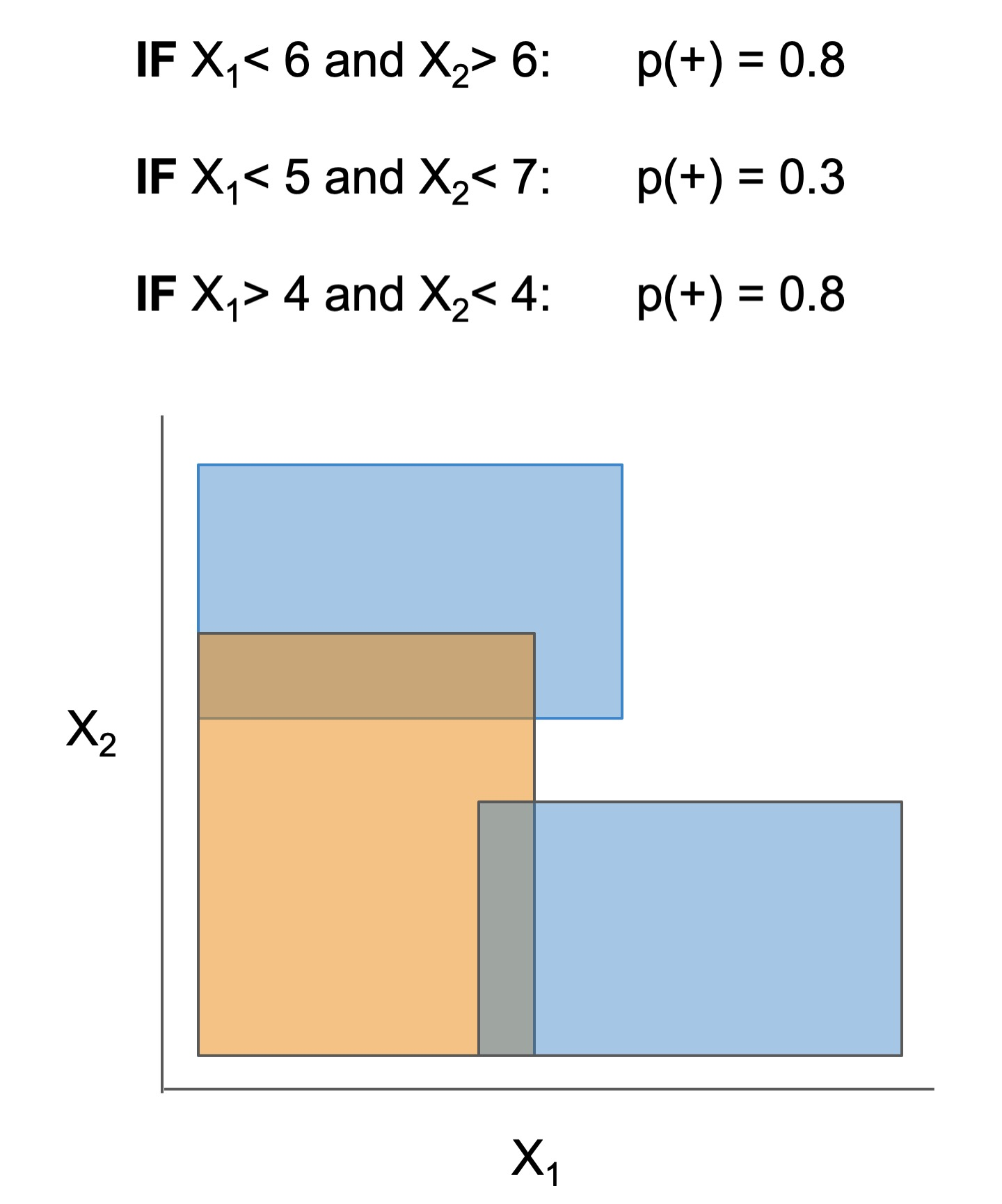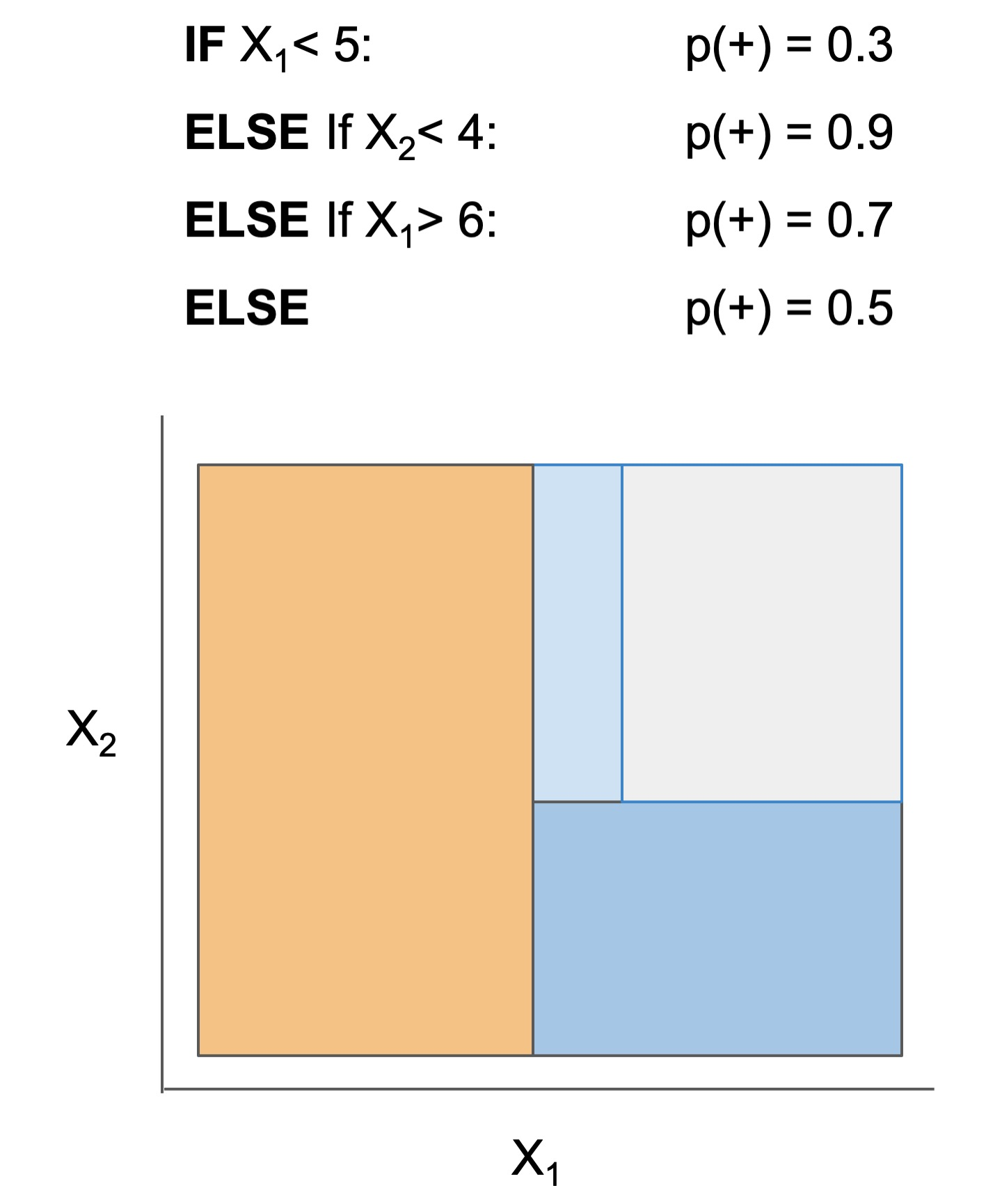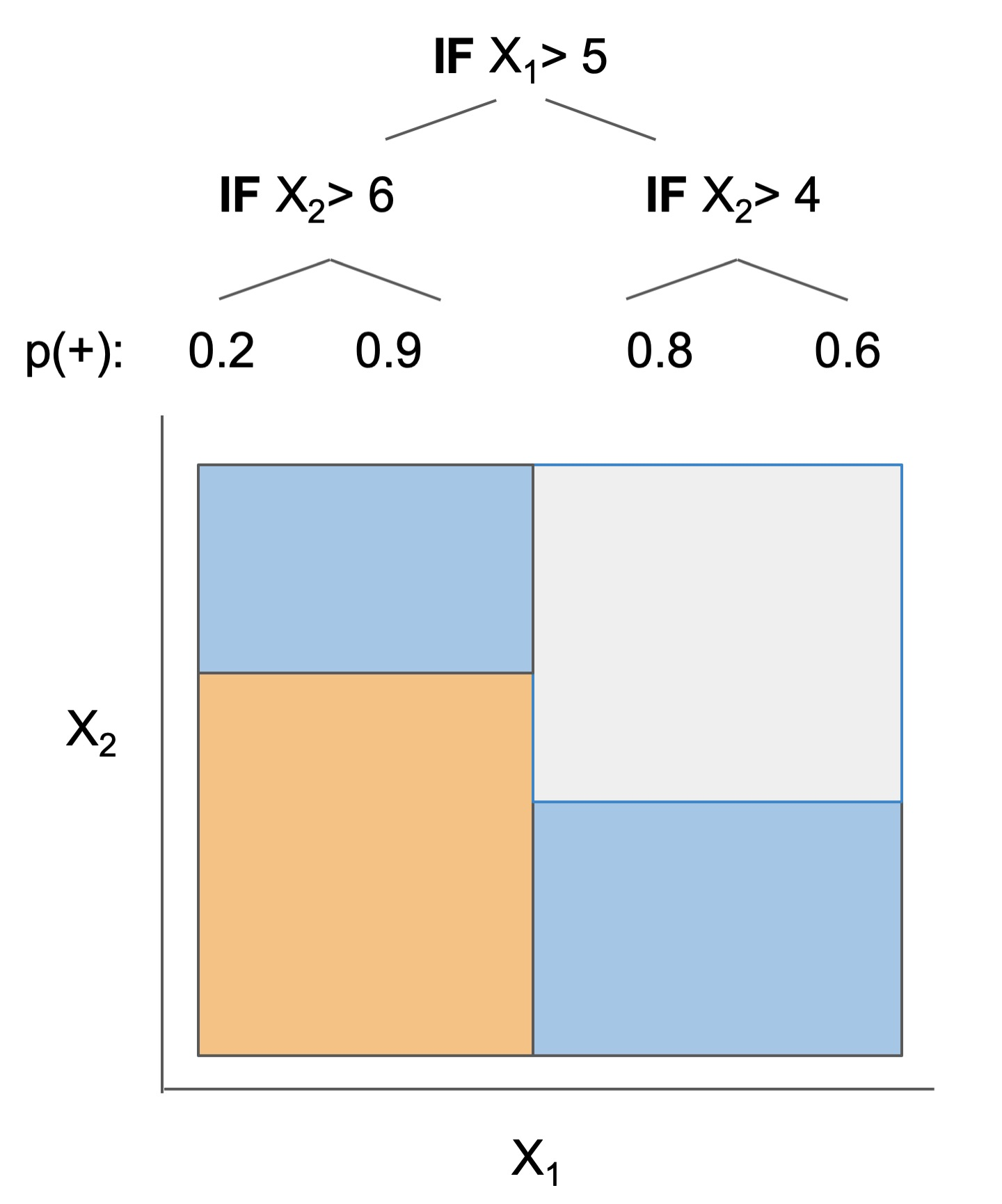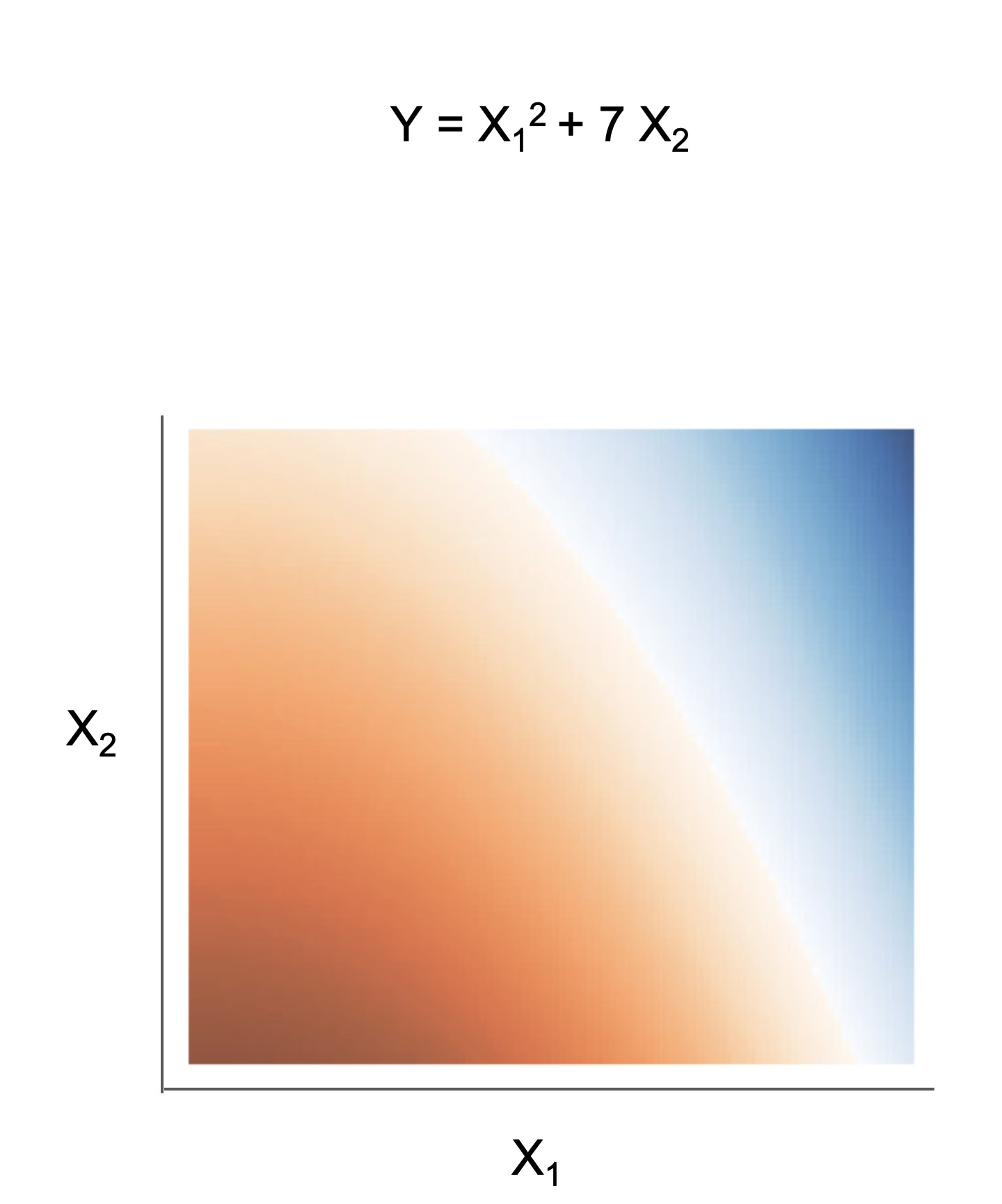Different models and algorithms vary not only in their final form but also in different choices made during modeling. In particular, many models differ in the 3 steps given by the table below.

ex. RuleFit and SkopeRules RuleFit and SkopeRules differ only in the way they prune rules: RuleFit uses a linear model whereas SkopeRules heuristically deduplicates rules sharing overlap.
ex. Bayesian rule lists and greedy rule lists Bayesian rule lists and greedy rule lists differ in how they select rules; bayesian rule lists perform a global optimization over possible rule lists while Greedy rule lists pick splits sequentially to maximize a given criterion.
ex. FPSkope and SkopeRules FPSkope and SkopeRules differ only in the way they generate candidate rules: FPSkope uses FPgrowth whereas SkopeRules extracts rules from decision trees.

See the docs for individual models for futher descriptions.

Rule candidate generation Rule selection Rule pruning / combination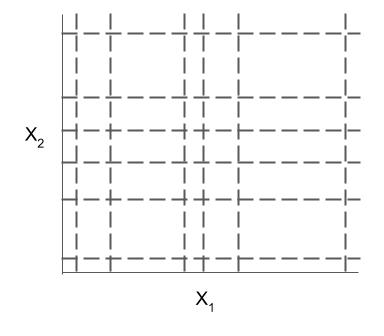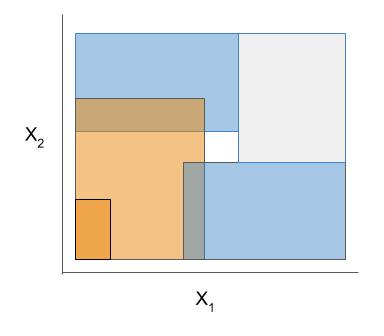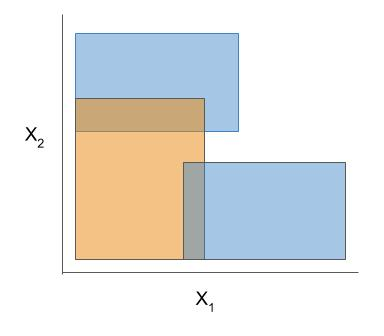The code here contains many useful and customizable functions for rule-based learning in the util folder. This includes functions / classes for rule deduplication, rule screening, and converting between trees, rulesets, and neural networks.

## Support for different tasks

Different models support different machine-learning tasks. Current support for different models is given below:

Model Binary classification Regression
Rulefit rule set ✔️ ✔️
Skope rule set ✔️
Boosted rule set ✔️
Bayesian rule list ✔️
Greedy rule list ✔️
OneR rule list ✔️
Optimal rule tree
Iterative random forest
Sparse integer linear model ✔️ ✔️

If it's useful for you, please cite the package using the below, but more importantly make sure to give authors of original methods / base implementations credit:

``````@software{
imodels2021,
title        = {{imodels: a python package for fitting interpretable models}},
publisher    = {Zenodo},
year         = 2021,
author       = {Chandan Singh and Keyan Nasseri and Bin Yu},
version      = {v0.2.8},
doi          = {10.5281/zenodo.4598963},
url          = {https://github.com/csinva/imodels}
}
``````